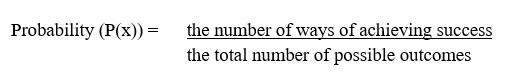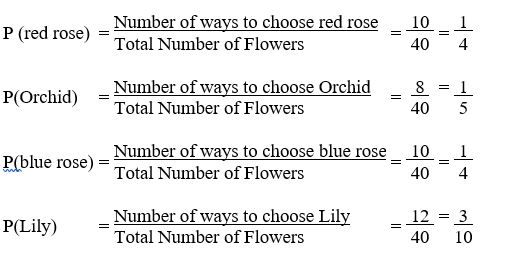### Probability Distribution Tutorial

• date 25th June, 2019 |
• by Prwatech |

# Probability Distribution Tutorial for Beginners

Probability Distribution Tutorial for beginners, Welcome to the world of statistics and probability in Data science using python. It is all about guesswork. Now, we are going to cover the introduction of the Probability distribution tutorial for Data science. Along with this, we will study various uses of it and formula to calculate with example.

### How Probability and statistics are used in Data Science?

Data science is based on statistics to predict or analyze the outcomes from data and statistics depends on probability distributions of data. Hence, it is essential to know about probability and its applications to work in Data science effectively.

Thinking of having a career as a Data analyst? Planning to go for a certification in Data science and related subjects but still not getting a conviction whether it is the right thing to do or not? Confused about whether to invest time in gaining knowledge of Data science or not? Such questions can give you sleepless nights, but by taking an advanced data science course from India’s largest E-Learning data science training institute in Bangalore, I am sure that you will get a lot of reasons to build a data science career.

## What is Probability distribution?

Probability is the measure of the likelihood that an event would occur in a Rando Experiment.

Its value lies between 0 to 1.

For example, the probability of flipping a coin and its being heads is ½, because there is 1 way of getting ahead and the total number of possible outcomes is 2 (head or tail). We write P(heads) = ½.

## Formula of Probability Distribution## Probability Distribution Example

A Bouquet of flowers contains 10 Red Roses, 8 Orchids, 10 Blue Roses and 12Lilies. If a single flower is chosen at random from the Bouquet, what is the probability of choosing a red rose, an Orchid flower, a blue rose, and a lily flower?

Probabilities:You are more likely to choose a Lily than any other color. You are least likely to choose a red rose or a blue rose.

I hope you have understood the basics of the Probability distribution tutorial for beginners and its formula with examples. Interested in learning more? Then Get enroll with Prwatechfor advanced Data science training in Bangalore with 100% placement assistance.

### Quick Support